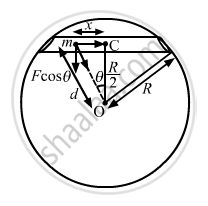Department of Pre-University Education, KarnatakaPUC Karnataka Science Class 11
Advertisement Remove all ads

# A Tunnel is Dug Along a Chord of the Earth at a Perpendicular Distance R/2 from the Earth'S Centre. the Wall of the Tunnel May Be Assumed to Be Frictionless. - Physics

Sum

A tunnel  is dug along a chord of the earth at a perpendicular distance R/2 from the earth's centre. The wall of the tunnel may be assumed to be frictionless. Find the force exerted by the wall on a particle of mass m when it is at a distance x from the centre of the tunnel.

Advertisement Remove all ads

#### Solution

Let d be distance of the particle from the centre of the Earth.$\text { Now }, d^2 = x^2 + \left( \frac{R^2}{4} \right) = \frac{4 x^2 + R^2}{4}$

$\Rightarrow d = \left( \frac{1}{2} \right)\sqrt{4 x^2 + R^2}$

Let M be the mass of the Earth and M' be the mass of the sphere of radius d.
Then we have :

$M = \left( \frac{4}{3} \right)\pi R^3 \rho$

$M^1 = \left( \frac{4}{3} \right)\pi d^3 \rho$

$\therefore \frac{M^1}{M} = \frac{d^3}{R^3}$

Gravitational force on the particle of mass m is given by

$F = \frac{G M^1 m}{d^2}$

$\Rightarrow F = \frac{G d^3 Mm}{R^3 d^2}$

$\Rightarrow F = \frac{GMm}{R^3}d$

∴ Normal force exerted by the wall, FN = F cos θ

$= \frac{GMmd}{R^3} \times \frac{R}{2d} = \frac{GMmd}{2 R^2}$

Is there an error in this question or solution?
Advertisement Remove all ads

#### APPEARS IN

HC Verma Class 11, 12 Concepts of Physics 1
Chapter 11 Gravitation
Q 12 | Page 226
Advertisement Remove all ads
Advertisement Remove all ads
Share
Notifications

View all notifications

Forgot password?